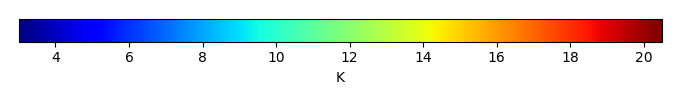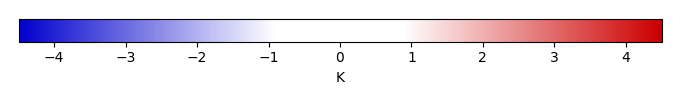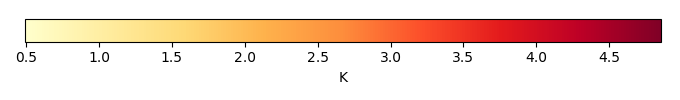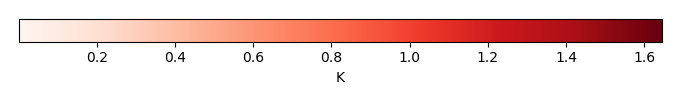# Mean State

Period Mean (original grids) [K]
Model Period Mean (intersection) [K]
Model Period Mean (complement) [K]
Benchmark Period Mean (intersection) [K]
Benchmark Period Mean (complement) [K]
Bias [K]
RMSE [K]
Phase Shift [months]
Bias Score 
RMSE Score 
Seasonal Cycle Score 
Spatial Distribution Score 
Interannual Variability Score 
Overall Score 
Benchmark [-] 10.8
CLM5PHSOFF [-] 11.6 11.8 6.15 10.9 8.01 0.937 1.47 0.579 0.423 0.533 0.921 0.975 0.507 0.649
CLM5PHSON [-] 12.0 12.0 7.44 10.9 8.01 0.911 1.41 0.537 0.424 0.556 0.925 0.974 0.497 0.656
Period Mean (original grids) [K]
Model Period Mean (intersection) [K]
Model Period Mean (complement) [K]
Benchmark Period Mean (intersection) [K]
Benchmark Period Mean (complement) [K]
Bias [K]
RMSE [K]
Phase Shift [months]
Bias Score 
RMSE Score 
Seasonal Cycle Score 
Spatial Distribution Score 
Interannual Variability Score 
Overall Score 
Benchmark [-] 13.8
CLM5PHSOFF [-] 14.5 14.6 7.01 13.8 11.0 0.791 1.26 0.449 0.743 0.742 0.955 0.979 0.772 0.822
CLM5PHSON [-] 14.6 14.7 9.22 13.8 11.0 0.822 1.26 0.504 0.734 0.749 0.948 0.986 0.765 0.822
Period Mean (original grids) [K]
Model Period Mean (intersection) [K]
Model Period Mean (complement) [K]
Benchmark Period Mean (intersection) [K]
Benchmark Period Mean (complement) [K]
Bias [K]
RMSE [K]
Phase Shift [months]
Bias Score 
RMSE Score 
Seasonal Cycle Score 
Spatial Distribution Score 
Interannual Variability Score 
Overall Score 
Benchmark [-] 12.8
CLM5PHSOFF [-] 13.0 13.5 4.93 12.8 9.07 0.701 1.29 0.403 0.574 0.690 0.951 0.979 0.691 0.763
CLM5PHSON [-] 13.9 14.0 6.81 12.8 9.07 0.800 1.35 0.410 0.549 0.696 0.951 0.981 0.687 0.760
Period Mean (original grids) [K]
Model Period Mean (intersection) [K]
Model Period Mean (complement) [K]
Benchmark Period Mean (intersection) [K]
Benchmark Period Mean (complement) [K]
Bias [K]
RMSE [K]
Phase Shift [months]
Bias Score 
RMSE Score 
Seasonal Cycle Score 
Spatial Distribution Score 
Interannual Variability Score 
Overall Score 
Benchmark [-] 9.39
CLM5PHSOFF [-] 9.19 9.53 5.00 9.41 7.83 0.0849 1.24 1.99 0.787 0.562 0.656 0.977 0.832 0.729
CLM5PHSON [-] 9.81 9.91 6.10 9.41 7.83 0.203 1.28 1.96 0.752 0.558 0.663 0.977 0.841 0.725
Period Mean (original grids) [K]
Model Period Mean (intersection) [K]
Model Period Mean (complement) [K]
Benchmark Period Mean (intersection) [K]
Benchmark Period Mean (complement) [K]
Bias [K]
RMSE [K]
Phase Shift [months]
Bias Score 
RMSE Score 
Seasonal Cycle Score 
Spatial Distribution Score 
Interannual Variability Score 
Overall Score 
Benchmark [-] 9.53
CLM5PHSOFF [-] 8.91 9.72 5.80 9.60 8.86 0.0681 1.69 0.915 0.232 0.488 0.875 0.968 0.763 0.636
CLM5PHSON [-] 10.2 10.4 7.01 9.60 8.86 0.116 1.72 0.900 0.224 0.492 0.876 0.963 0.763 0.635
Period Mean (original grids) [K]
Model Period Mean (intersection) [K]
Model Period Mean (complement) [K]
Benchmark Period Mean (intersection) [K]
Benchmark Period Mean (complement) [K]
Bias [K]
RMSE [K]
Phase Shift [months]
Bias Score 
RMSE Score 
Seasonal Cycle Score 
Spatial Distribution Score 
Interannual Variability Score 
Overall Score 
Benchmark [-] 10.7
CLM5PHSOFF [-] 10.9 10.9 5.53 10.7 8.60 0.263 1.08 1.21 0.792 0.641 0.811 0.977 0.938 0.800
CLM5PHSON [-] 11.2 11.2 6.00 10.7 8.60 0.480 1.14 1.16 0.756 0.641 0.821 0.989 0.944 0.799
Period Mean (original grids) [K]
Model Period Mean (intersection) [K]
Model Period Mean (complement) [K]
Benchmark Period Mean (intersection) [K]
Benchmark Period Mean (complement) [K]
Bias [K]
RMSE [K]
Phase Shift [months]
Bias Score 
RMSE Score 
Seasonal Cycle Score 
Spatial Distribution Score 
Interannual Variability Score 
Overall Score 
Benchmark [-] 11.7
CLM5PHSOFF [-] 11.8 12.0 5.38 11.8 9.57 0.205 1.07 0.674 0.742 0.639 0.907 0.999 0.916 0.807
CLM5PHSON [-] 12.2 12.3 6.69 11.8 9.57 0.292 1.08 0.681 0.737 0.639 0.905 0.999 0.916 0.806
Period Mean (original grids) [K]
Model Period Mean (intersection) [K]
Model Period Mean (complement) [K]
Benchmark Period Mean (intersection) [K]
Benchmark Period Mean (complement) [K]
Bias [K]
RMSE [K]
Phase Shift [months]
Bias Score 
RMSE Score 
Seasonal Cycle Score 
Spatial Distribution Score 
Interannual Variability Score 
Overall Score 
Benchmark [-] 11.1
CLM5PHSOFF [-] 11.2 11.7 5.36 11.2 8.50 0.532 1.23 0.484 0.677 0.733 0.942 0.971 0.834 0.815
CLM5PHSON [-] 12.1 12.2 6.83 11.2 8.50 0.602 1.27 0.497 0.661 0.733 0.940 0.973 0.835 0.813
Period Mean (original grids) [K]
Model Period Mean (intersection) [K]
Model Period Mean (complement) [K]
Benchmark Period Mean (intersection) [K]
Benchmark Period Mean (complement) [K]
Bias [K]
RMSE [K]
Phase Shift [months]
Bias Score 
RMSE Score 
Seasonal Cycle Score 
Spatial Distribution Score 
Interannual Variability Score 
Overall Score 
Benchmark [-] 8.50
CLM5PHSOFF [-] 8.31 8.51 5.01 8.51 7.45 -0.00427 1.12 1.09 0.816 0.588 0.819 0.972 0.804 0.765
CLM5PHSON [-] 8.59 8.67 5.72 8.51 7.45 0.0319 1.11 0.752 0.816 0.592 0.882 0.969 0.807 0.776
Period Mean (original grids) [K]
Model Period Mean (intersection) [K]
Model Period Mean (complement) [K]
Benchmark Period Mean (intersection) [K]
Benchmark Period Mean (complement) [K]
Bias [K]
RMSE [K]
Phase Shift [months]
Bias Score 
RMSE Score 
Seasonal Cycle Score 
Spatial Distribution Score 
Interannual Variability Score 
Overall Score 
Benchmark [-] 13.7
CLM5PHSOFF [-] 13.9 14.3 6.37 13.8 10.4 0.567 1.25 0.374 0.607 0.701 0.964 0.928 0.912 0.802
CLM5PHSON [-] 14.7 14.8 7.39 13.8 10.4 0.595 1.25 0.385 0.603 0.706 0.964 0.932 0.913 0.804
Period Mean (original grids) [K]
Model Period Mean (intersection) [K]
Model Period Mean (complement) [K]
Benchmark Period Mean (intersection) [K]
Benchmark Period Mean (complement) [K]
Bias [K]
RMSE [K]
Phase Shift [months]
Bias Score 
RMSE Score 
Seasonal Cycle Score 
Spatial Distribution Score 
Interannual Variability Score 
Overall Score 
Benchmark [-] 8.57
CLM5PHSOFF [-] 8.74 9.06 5.45 8.60 7.69 0.447 1.28 0.644 0.657 0.660 0.916 0.950 0.875 0.786
CLM5PHSON [-] 9.40 9.50 6.61 8.60 7.69 0.520 1.31 0.649 0.639 0.666 0.915 0.943 0.872 0.784
Period Mean (original grids) [K]
Model Period Mean (intersection) [K]
Model Period Mean (complement) [K]
Benchmark Period Mean (intersection) [K]
Benchmark Period Mean (complement) [K]
Bias [K]
RMSE [K]
Phase Shift [months]
Bias Score 
RMSE Score 
Seasonal Cycle Score 
Spatial Distribution Score 
Interannual Variability Score 
Overall Score 
Benchmark [-] 11.6
CLM5PHSOFF [-] 11.4 12.2 6.90 11.8 7.98 0.396 1.25 0.659 0.641 0.642 0.909 0.994 0.799 0.771
CLM5PHSON [-] 12.0 12.6 7.84 11.8 7.98 0.472 1.27 0.655 0.629 0.646 0.910 0.994 0.799 0.771
Period Mean (original grids) [K]
Model Period Mean (intersection) [K]
Model Period Mean (complement) [K]
Benchmark Period Mean (intersection) [K]
Benchmark Period Mean (complement) [K]
Bias [K]
RMSE [K]
Phase Shift [months]
Bias Score 
RMSE Score 
Seasonal Cycle Score 
Spatial Distribution Score 
Interannual Variability Score 
Overall Score 
Benchmark [-] 14.0
CLM5PHSOFF [-] 14.7 15.1 6.64 14.0 11.1 1.04 1.54 0.528 0.454 0.619 0.931 0.967 0.731 0.721
CLM5PHSON [-] 15.3 15.4 8.58 14.0 11.1 1.09 1.58 0.572 0.442 0.617 0.925 0.970 0.733 0.717
Period Mean (original grids) [K]
Model Period Mean (intersection) [K]
Model Period Mean (complement) [K]
Benchmark Period Mean (intersection) [K]
Benchmark Period Mean (complement) [K]
Bias [K]
RMSE [K]
Phase Shift [months]
Bias Score 
RMSE Score 
Seasonal Cycle Score 
Spatial Distribution Score 
Interannual Variability Score 
Overall Score 
Benchmark [-] 8.40
CLM5PHSOFF [-] 8.06 8.35 5.12 8.41 7.44 -0.0839 1.07 0.906 0.799 0.573 0.857 0.977 0.904 0.781
CLM5PHSON [-] 8.58 8.67 5.85 8.41 7.44 -0.0175 1.07 0.851 0.794 0.573 0.867 0.971 0.906 0.781
Period Mean (original grids) [K]
Model Period Mean (intersection) [K]
Model Period Mean (complement) [K]
Benchmark Period Mean (intersection) [K]
Benchmark Period Mean (complement) [K]
Bias [K]
RMSE [K]
Phase Shift [months]
Bias Score 
RMSE Score 
Seasonal Cycle Score 
Spatial Distribution Score 
Interannual Variability Score 
Overall Score 
Benchmark [-] 13.3
CLM5PHSOFF [-] 12.6 13.4 7.19 13.4 10.3 -0.0721 1.51 0.511 0.581 0.618 0.939 0.974 0.804 0.756
CLM5PHSON [-] 14.0 14.3 8.96 13.4 10.3 -0.00928 1.53 0.501 0.575 0.620 0.942 0.974 0.803 0.755
Period Mean (original grids) [K]
Model Period Mean (intersection) [K]
Model Period Mean (complement) [K]
Benchmark Period Mean (intersection) [K]
Benchmark Period Mean (complement) [K]
Bias [K]
RMSE [K]
Phase Shift [months]
Bias Score 
RMSE Score 
Seasonal Cycle Score 
Spatial Distribution Score 
Interannual Variability Score 
Overall Score 
Benchmark [-] 12.2
CLM5PHSOFF [-] 11.8 12.0 7.40 12.2 9.97 -0.161 0.936 0.819 0.758 0.658 0.874 0.920 0.944 0.802
CLM5PHSON [-] 12.2 12.2 9.22 12.2 9.97 -0.0749 0.948 0.838 0.752 0.656 0.871 0.911 0.943 0.798
Period Mean (original grids) [K]
Model Period Mean (intersection) [K]
Model Period Mean (complement) [K]
Benchmark Period Mean (intersection) [K]
Benchmark Period Mean (complement) [K]
Bias [K]
RMSE [K]
Phase Shift [months]
Bias Score 
RMSE Score 
Seasonal Cycle Score 
Spatial Distribution Score 
Interannual Variability Score 
Overall Score 
Benchmark [-] 11.0
CLM5PHSOFF [-] 11.0 11.1 5.95 11.1 7.54 0.0242 1.05 0.767 0.775 0.667 0.891 0.998 0.944 0.823
CLM5PHSON [-] 11.4 11.4 7.11 11.1 7.54 0.174 1.05 0.737 0.762 0.676 0.895 0.997 0.945 0.825
Period Mean (original grids) [K]
Model Period Mean (intersection) [K]
Model Period Mean (complement) [K]
Benchmark Period Mean (intersection) [K]
Benchmark Period Mean (complement) [K]
Bias [K]
RMSE [K]
Phase Shift [months]
Bias Score 
RMSE Score 
Seasonal Cycle Score 
Spatial Distribution Score 
Interannual Variability Score 
Overall Score 
Benchmark [-] 15.3
CLM5PHSOFF [-] 14.8 14.9 6.95 15.3 11.3 -0.457 1.26 0.444 0.751 0.658 0.947 0.999 0.933 0.824
CLM5PHSON [-] 15.1 15.1 9.64 15.3 11.3 -0.320 1.20 0.455 0.769 0.663 0.946 0.999 0.931 0.828
Period Mean (original grids) [K]
Model Period Mean (intersection) [K]
Model Period Mean (complement) [K]
Benchmark Period Mean (intersection) [K]
Benchmark Period Mean (complement) [K]
Bias [K]
RMSE [K]
Phase Shift [months]
Bias Score 
RMSE Score 
Seasonal Cycle Score 
Spatial Distribution Score 
Interannual Variability Score 
Overall Score 
Benchmark [-] 10.2
CLM5PHSOFF [-] 10.9 11.0 5.71 10.2 9.50 0.787 1.14 0.382 0.498 0.640 0.952 0.988 0.473 0.698
CLM5PHSON [-] 11.1 11.1 7.07 10.2 9.50 0.792 1.12 0.433 0.493 0.656 0.948 0.991 0.463 0.701
Period Mean (original grids) [K]
Model Period Mean (intersection) [K]
Model Period Mean (complement) [K]
Benchmark Period Mean (intersection) [K]
Benchmark Period Mean (complement) [K]
Bias [K]
RMSE [K]
Phase Shift [months]
Bias Score 
RMSE Score 
Seasonal Cycle Score 
Spatial Distribution Score 
Interannual Variability Score 
Overall Score 
Benchmark [-] 11.7
CLM5PHSOFF [-] 12.0 12.3 6.02 11.7 9.16 0.567 0.964 0.374 0.635 0.682 0.955 0.984 0.636 0.762
CLM5PHSON [-] 12.4 12.5 7.16 11.7 9.16 0.663 1.02 0.367 0.600 0.687 0.955 0.985 0.632 0.758
Period Mean (original grids) [K]
Model Period Mean (intersection) [K]
Model Period Mean (complement) [K]
Benchmark Period Mean (intersection) [K]
Benchmark Period Mean (complement) [K]
Bias [K]
RMSE [K]
Phase Shift [months]
Bias Score 
RMSE Score 
Seasonal Cycle Score 
Spatial Distribution Score 
Interannual Variability Score 
Overall Score 
Benchmark [-] 12.5
CLM5PHSOFF [-] 12.6 13.0 6.46 12.6 7.59 0.379 1.42 0.824 0.607 0.613 0.899 0.978 0.741 0.742
CLM5PHSON [-] 13.7 13.7 8.63 12.6 7.59 0.457 1.44 0.845 0.595 0.615 0.897 0.976 0.743 0.740

# Temporally integrated period mean

BENCHMARK MEANMODEL MEANBIASBIAS SCORERMSERMSE SCOREBENCHMARK INTERANNUAL VARIABILITYMODEL INTERANNUAL VARIABILITYINTERANNUAL VARIABILITY SCOREBENCHMARK MAX MONTHMODEL MAX MONTHDIFFERENCE IN MAX MONTHSEASONAL CYCLE SCORESPATIAL TAYLOR DIAGRAMMODEL COLORS# Spatially integrated regional mean

MODEL COLORSREGIONAL MEANANNUAL CYCLEMONTHLY ANOMALYANNUAL CYCLE# All Models

BenchmarkCLM5PHSOFFCLM5PHSON# Data Information

creation_date: Fri Feb 8 13:55:45 PST 2019

history: 2018-01-15: download from https://crudata.uea.ac.uk/cru/data/hrg/cru_ts_4.01/cruts.1709081022.v4.01/ 2019-02-06: converted and created to netCDF4 for ILAMB package

original_resolution: 0.5x0.5

resolution: 0.5x0.5

original_frequency: mon

frequency: mon

references: Harris et al., 2014, Int. J. Climatol., doi:10.1002/joc.3711

institution: Original Data held at British Atmospheric Data Centre, RAL, UK.

source: Run ID = 1709081022. Data generated from:dtr.1708250000.dtb

title: CRU TS4.01 Diurnal Temperature Range

Conventions: CF-1.7